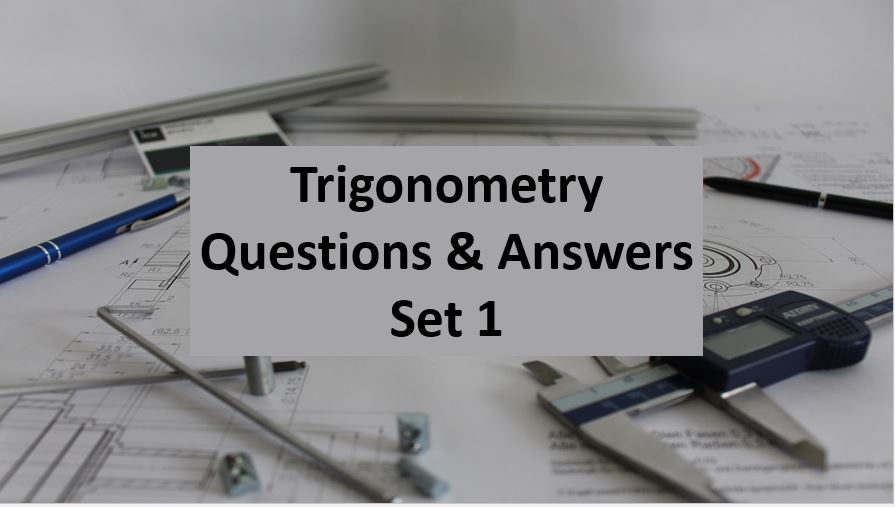Monday, September 27, 2021
Home > Question and Answer > Trigonometry Question And Answer Set 1

# Trigonometry Question And Answer Set 1# Trigonometry Question and Answer Set 1

Hi students, welcome to Amans Maths Blogs (AMB). On this post, you will get the Trigonometry Question and Answer Set 1 is the collection of some important questions. Practice these questions for SSC CGL CHSL CAT NTSE NSEJS exams etc. It will help you to practice the questions on the topics of trigonometry based questions with answers.

Trigonometry Question and Answer Set 1: Ques No 1

If tan= cot, then the value of sin+ cosis

Options:

A. 0

B. 1

C. 2

D. Root(2)

Trigonometry Question and Answer Set 1: Ques No 2

If tan+ cot= 2, then the value of tan200+ cot100is

Options:

A. Root(3)

B. 1

C. 2

D. Root(2)

Trigonometry Question and Answer Set 1: Ques No 3

In a ΔABC, the angle B =  π/3  and the angle C =  π/4  and D divides BC internally in the ratio 1 : 3 then (sin ∠BAD) / (sin ∠CAD) is equal to

Options:

A. 1/Root(6)

B. 1/Root(2)

C. 1/Root(3)

D. 1/Root(5)

Trigonometry Question and Answer Set 1: Ques No 4

If sec+ tan= 2, then the value of cosis

Options:

A. 3/5

B. 4/5

C. 5/3

D. 5/4

Trigonometry Question and Answer Set 1: Ques No 5

The smallest value of r for which 3sinx + 4cosx + r is always greater than or equal to 0 is

Options:

A. 3

B. 4

C. 5

D. -5

Trigonometry Question and Answer Set 1: Ques No 6

If tanϕ + sinϕ = m, tanϕ − sinϕ = n, Find the value of m2 – nis

Options:

A. 2Root(mn)

B. 4Root(mn)

C. m – n

D. 2mn

Trigonometry Question and Answer Set 1: Ques No 7

If tan ϕ = 3/4, then the value of (1 – cos ϕ)/(1 + cos ϕ) is

Options:

A. 1/3

B. 1/9

C. 2/5

D. 3/8

Trigonometry Question and Answer Set 1: Ques No 8

The value of (1 + cos π/8) (1 + cos 3π/8) (1 + cos 5π/8)(1 + cos 7π/8) is

Options:

A. 1/2

B. 1/4

C. 1/5

D. 1/8

Trigonometry Question and Answer Set 1: Ques No 9

If cosA = cosBcosC, then the value of tan(A + B)/2 + tan(A – B)/2 is

Options:

A. tan2A/2

B. tan2B/2

C. tan2C/2

D. cot2A/2